# simulate inverting and non inverting amplifiers in PSpice : tutorial 7

In this tutorial I will explain you the working of an operation amplifier. Both inverting and non-inverting amplifiers are explained and simulated using PSPICE. At the start a brief and concise introduction of inverting and non-inverting amplifiers is provided with the explanation of the output they will show. After that both the circuits are simulated using PSPICE and the result are compared with the theoretical discussion provided (which should be same). At the end of the tutorial you are provided with an exercise to do it by yourself, and in the next tutorials I will assume that you have done those exercises and I will not explain the concept regarding them

### Introduction to inverting and non-Inverting amplifiers

Amplifier as the named implies are used for the amplification of a signal. The signal can be amplified either in positive direction or in negative direction. Two types of amplifiers are very common in electronics i.e.

• Inverting amplifier
• Non-inverting amplifier

### Inverting amplifier simulation PSPICE

An inverting amplifier is one which the output is given as feedback to the negative terminal of the operation amplifier. The output of the inverting amplifier is the inverted form of the input signal as the name implies multiplied by the gain factor and is 180 degrees out of phase in case of sinusoidal input. A simple circuit diagram of an inverting amplifier is shown in the figure below,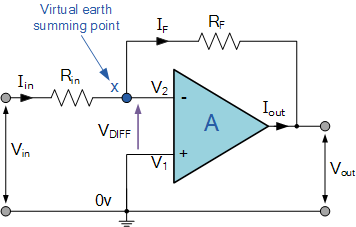Figure 1: Inverting amplifier

The output of an inverting amplifier is given in the figure below,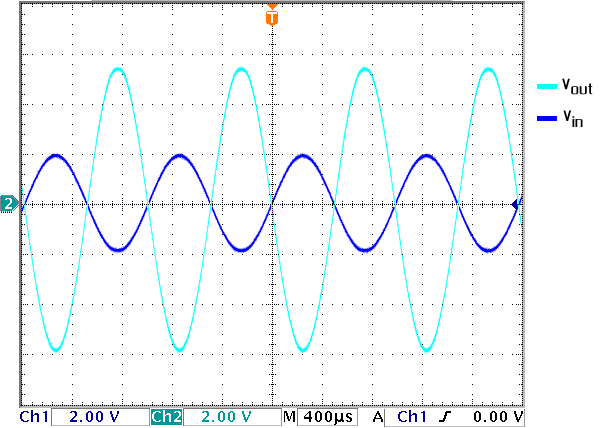Figure 2: Output of inverting amplifier

And the formula to calculate the gain of an inverting amplifier is

### Non-Inverting amplifier Simulation PSPICE

A non-inverting amplifier is one which the output is given as feedback to the negative terminal of the operation amplifier, however the input is supplied through the positive terminal. The output of the inverting amplifier is same as the input signal multiplied by the gain factor and is in phase in case of sinusoidal input. A simple circuit diagram of an non-inverting amplifier is shown in the figure below,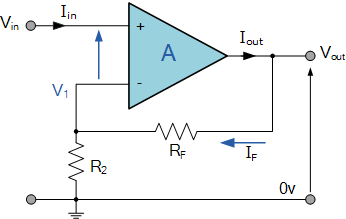Figure 1: Inverting amplifier

The output of a non-inverting amplifier is given in the figure below,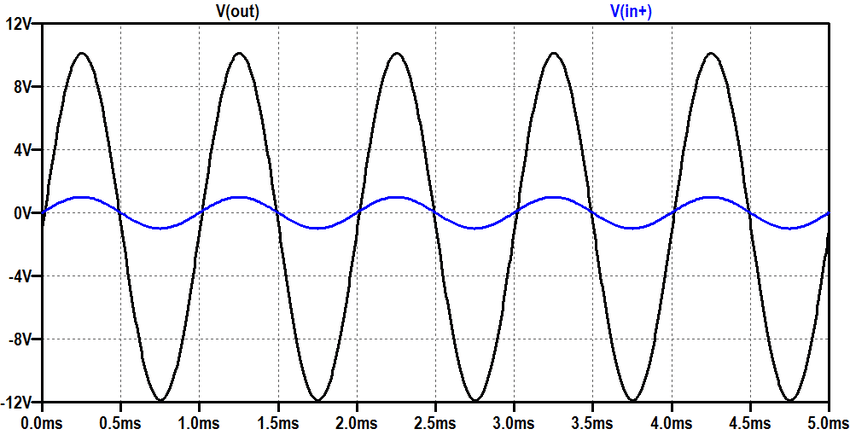Figure 2: Output of non-inverting amplifier

And the formula to calculate the gain of a non-inverting amplifier is

### Explanation with example of simulation in PSPICE

Figure 3: Opening Pspice

Figure 4: Saving schematic

Figure 5: Getting new part

Figure 6: Placing opamp

Figure 7: Placing resistor

Figure 8: Placing sin

Figure 9: Placing ground

Figure 10: Placed components

Figure 11: Drawing wire

Figure 12: Complete circuit diagram

Figure 13: Voltage marker

Figure 14: Placed voltage marker

Figure 15: Sin attributes

Figure 16: Analysis setup

Figure 17: Transient properties

Figure 18: Labeling the wires

Figure 19: Simulating

Figure 20: Output on an inverting amplifier

• The output of the circuit is in accordance with the output expected. An inverting amplifier as the name suggests inverts the voltage applied at the input side of the operational amplifier, and the magnitude of the output is amplified according to the gain of the amplifier adjusted with the resistors connected with it. As the input supply is connected to the inverting side of the amplifier i.e. the negative side of the amplifier hence the voltage at the output is 180 degrees out of phase of the input. The amplitude of the voltage can be increased or decreased according to the requirement using the gain formula provided in the introduction portion.
• Lets’ now move towards the non-inverting amplifier, the circuit diagram of a non-inverting amplifier, simulated in the schematic of PSPICE is shown in the figure below,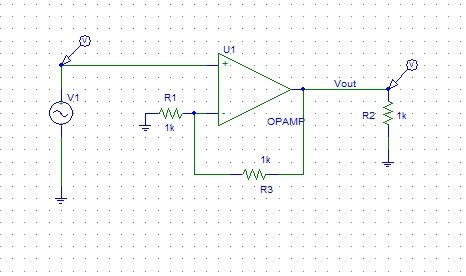Figure 21: Non-inverting amplifier

Figure 22: Non-Inverting amplifier

• As discussed in the introduction part, the output of the non-inverting amplifier is in phase with the input and its amplitude is increased or decreased according to the gain of the amplifier, the formula of which is given in the introduction portion. The simulation of the non-inverting amplifier is left for the reader as an exercise.

Exercise:

• Simulate the non-inverting amplifier circuit using the results and circuit diagram provided above.

<< Previous tutorial                                             Next tutorial>>